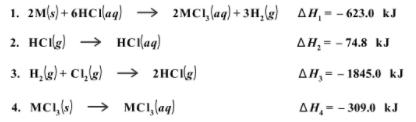Problem: Consider these reactions, where M represents a generic metal. Use the information below to determine the enthalpy of the following reaction. 2M(s) + 3Cl2(g) → 2MCl3(s)

99% (97 ratings)
Problem Details

Consider these reactions, where M represents a generic metal. Use the information below to determine the enthalpy of the following reaction. 2M(s) + 3Cl2(g) → 2MCl3(s)